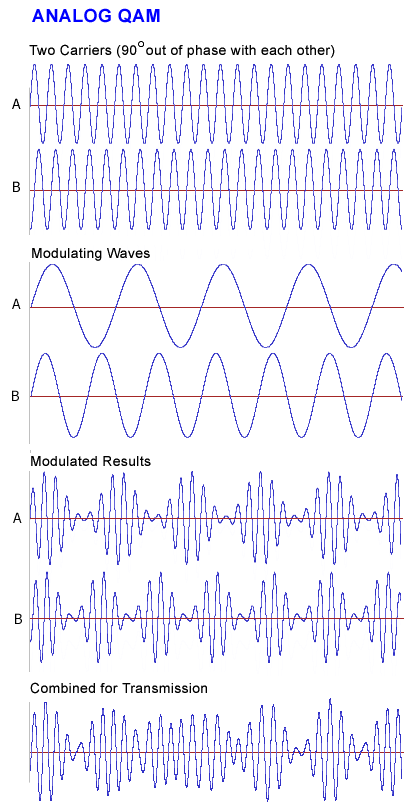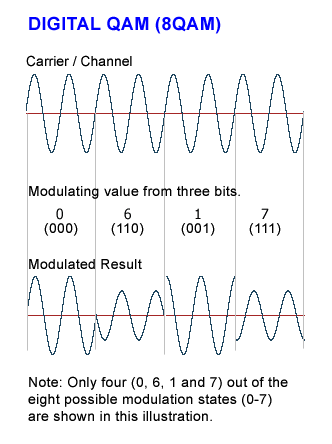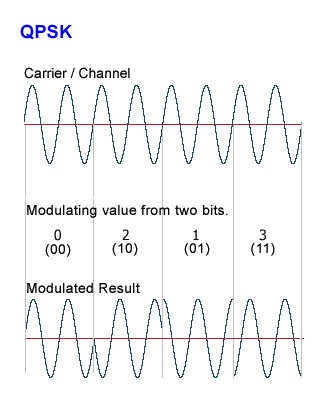Also found in: Dictionary, Thesaurus, Acronyms, Wikipedia.

in astronomy, arrangement of two celestial bodies at right angles to each other as viewed from a reference point. If the reference point is the earth and the sun is one of the bodies, a planet is in quadrature when its elongationelongation,
in astronomy, the angular distance between two points in the sky as measured from a third point. The elongation of a planet is usually measured as the angular distance from the sun to the planet as measured from the earth.
is 90°. As viewed from the earth, the half-moon is in quadrature to the sun. The inferior planets (Mercury and Venus) never reach quadrature; the superior planets each have two points of quadrature in their orbits.

(kwod -ră-cher) The position, or aspect, of a body in the Solar System when its elongation from the Sun, as measured from the Earth, is 90°, i.e. when the angle Sun–Earth–body is a right angle.
Collins Dictionary of Astronomy © Market House Books Ltd, 2006

[′kwä·drə·chər]
(astronomy)
The right-angle physical alignment of the sun, moon, and earth.
(mathematics)
The construction of a square whose area is equal to that of a given surface.
The process of calculating a definite integral.
(physics)
State of being separated in phase by 90°, or one quarter-cycle. Also known as phase quadrature.
McGraw-Hill Dictionary of Scientific & Technical Terms, 6E, Copyright © 2003 by The McGraw-Hill Companies, Inc.

QAM

(1) (Quality Assessment Measurement) A system used to measure and analyze voice transmission.

(2) (Quantum Abstract Machine) An interface from Rigetti Computing for running programs in a hybrid classical/quantum computer environment. See QVM and quantum computing.

(3) (Quadrature Amplitude Modulation) A modulation technique that employs both phase modulation (PM) and amplitude modulation (AM). Widely used to transmit digital signals such as digital cable TV and cable Internet service, QAM is also used as the modulation technique in orthogonal frequency division multiplexing (see OFDM). The "quadrature" comes from the fact that the phase modulation states are 90 degrees apart from each other.

Analog QAM
Analog QAM uses two carriers 90 degrees out of phase with each other. Each carrier is modulated by an analog signal, and the resulting modulated waves are combined (see example below).

Digital QAM
In digital QAM, the number of modulation states determines how the digital signal is split up. For example, in 8QAM, each three bits of input alters the phase and amplitude of the carrier to derive eight unique modulation states (see example below).

In 16QAM, 32QAM, 64QAM, 128QAM, 256QAM, 512QAM and 1024QAM, from four to 10 bits generate from 16 to 1,024 modulation states respectively. See modulation and binary values.

Analog QAMAnalog QAM modulates two carriers 90 degrees out of phase with each from two analog input streams. The modulated carriers are combined and transmitted.

Digital QAM (8QAM)In 8QAM, three input bits generate eight modulation states using four phase angles on 90 degree boundaries and two amplitudes (4 phases X 2 amplitudes = 8 states). Digital modulation greater than 8QAM is difficult to visualize.

Quadrature PSK (QPSK)QPSK is like 4QAM without amplitude modulation. QPSK uses four phase angles to represent each two bits of input; however, the amplitude remains constant.
Copyright © 1981-2019 by The Computer Language Company Inc. All Rights reserved. THIS DEFINITION IS FOR PERSONAL USE ONLY. All other reproduction is strictly prohibited without permission from the publisher.
References in periodicals archive ?
(2) For a monotone function f that has a singularity at x = a, it is clear that the closed type quadrature [Q.sup.2.sub.n](f) = 1/2[a.sub.n1] f ([u.sub.n1]) + [[summation].sup.n.sub.k=2] [a.sub.nk]/2 (f ([u.sub.n,k-1]) + f ([u.sub.nk])) is better than the open type quadrature [Q.sup.1.sub.n](f).
Each of the above three quadrature rules (6), (7) & (8) is of precision five.
Spectral sensitivity of power amplifiers to quadrature modulator misalignment IEEE Transactions on Vehicular Technology, 41(4).
Then the Gauss quadrature is given by the unique n-node quadrature formula which matches the first 2n moments of the Riemann-Stieltjes integral (3.1).
In the paper, it is assumed, that the quadrature booster is represented by the model to be shown in Fig.
The quadrature oscillator had been tuned by control voltage (V) in a linear range (band-spread) of 0.5 [less than or equal to] [f.sub.o] (MHZ) [less than or equal to] 9.9 at low THD.
where [w.sub.l] are positive weights, [[xi].sub.1] are the coordinates of points, and [N.sub.max] is the number of points in the quadrature formula corresponding to the spectral element of the order N.
For 3D transport calculation, the level symmetric quadrature sets (LS or L[Q.sub.N]) developed by Lathrop and Carlson are still the most widely and commonly used sets .
where [t.sub.j] denote the quadrature points, which can be solved from the solutions of the following m-order Chebyshev-Laguerre polynomial.
[S.sup.(0).sub.oi] (t) and [S.sup.(0).sub.oq](t) denote the in-phase and the quadrature components of the expected baseband signal; [s.sup.(j).sub.pi] (t) and [s.sup.(j).sub.pq] (t) represent the in-phase and the quadrature components of the interfering baseband signal from the j th cell.

Site: Follow: Share:
Open / Close